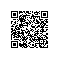# 掌握Javascript面试：什么是闭包？

“如果你不能回答这个问题，你就是一个初级开发人员。我不管你工作了几年。”

### 使用闭包（实例）

“程序之于接口，而不是实现”

const getSecret = (secret) => {
return {
get: () => secret
};
};

test('Closure for object privacy.', assert => {
const expected = 1;
const obj = getSecret(1);

const actual = obj.get();

try {
assert.ok(secret, 'This throws an error.');
} catch (e) {
assert.ok(true, The secret var is only available
to privileged methods.);
}

assert.equal(actual, expected, msg);
assert.end();
});


const secret = msg => () => msg;

// Secret - creates closures with secret messages.
// https://gist.github.com/ericelliott/f6a87bc41de31562d0f9
// https://jsbin.com/hitusu/edit?html,js,output

// secret(msg: String) => getSecret() => msg: String
const secret = (msg) => () => msg;

test('secret', assert => {
const msg = 'secret() should return a function that returns the passed secret.';

const theSecret = 'Closures are easy.';
const mySecret = secret(theSecret);

const actual = mySecret();
const expected = theSecret;

assert.equal(actual, expected, msg);
assert.end();
});


partialApply(targetFunction: Function, ...fixedArgs: Any[]) =>
functionWithFewerParams(...remainingArgs: Any[])


const add = (a, b) => a + b;


const add10 = partialApply(add, 10);


// Generic Partial Application Function
// https://jsbin.com/biyupu/edit?html,js,output
// https://gist.github.com/ericelliott/f0a8fd662111ea2f569e

// partialApply(targetFunction: Function, ...fixedArgs: Any[]) =>
//   functionWithFewerParams(...remainingArgs: Any[])
const partialApply = (fn, ...fixedArgs) => {
return function (...remainingArgs) {
return fn.apply(this, fixedArgs.concat(remainingArgs));
};
};
const msg = 'partialApply() should partially apply functions'
const add = (a, b) => a + b;
const expected = 15;

assert.equal(actual, expected, msg);
});使用钉钉扫一扫加入圈子
+ 订阅